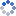加载中…聂远• 博客等级：• 博客积分：0
• 博客访问：4,947,673
• 关注人气：5,750
• 获赠金笔：0支
• 赠出金笔：0支
• 荣誉徽章：

## 南非行手记1——世界杯在路上(2010-06-15 17:17:48)

### 娱乐………………………………………………………………………………………………………………………………………………………………………………………………………………………………………………………………………………………………………………………………空中……翻滚的云像激动的心，其实我们都在吃吃睡睡……^^^^^^^^^^^^^^^^^^^^^^^^^^^^^^^^^^^^^^^^^^^^^^^^^^^^^^^^^^^^^^^^^^^^^^^^^^^^^^^^^^^^^^^^^^^^^^12个12小时之后，睁眼看见开普敦！0